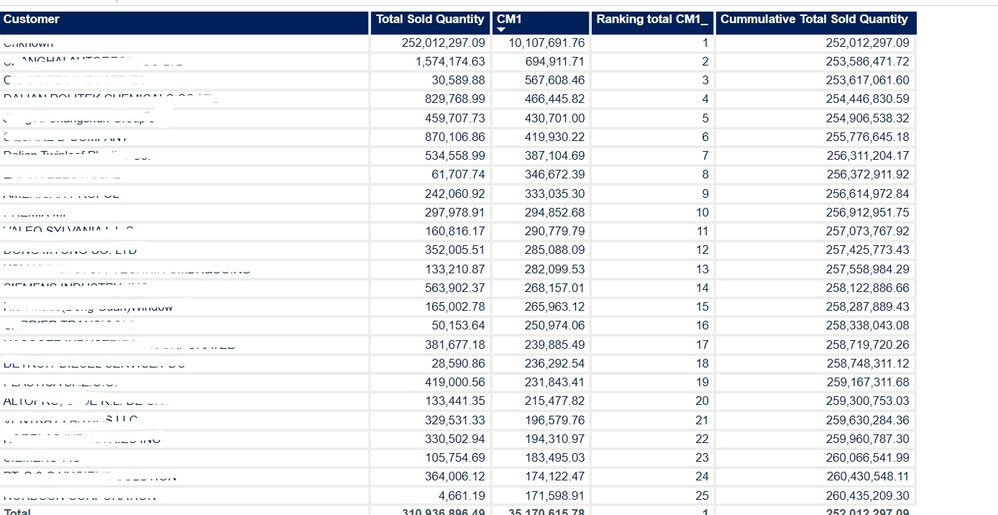cancel
Showing results for
Did you mean:Frequent Visitor

## Cumulative by rank is very slow in power bi

Hi All,

I am trying to calculate cumulative total by rank, my scneriao is I have a metric table where I got "total quantity" and "CM1"as a measure. first I want to rank customer by "CM1" and then based on ranking I want to calculate cumulative total sold quantity.

However I have been able to achived this calculation but its taking very long time to load the table visuals, it takes alomost 2 to 5 mins. and that is because in the table it has more than 21 thousand rows.

Hers is the measure calcuation -

Cummulative Total Sold Quantity =
VAR CurrentRank = [Ranking total CM1_]
VAR SummarizeCustomer =
SUMMARIZE(
ALLSELECTED('Customer Parameter_'),'Customer Parameter_'[Customer])
VAR CustomerSales =
ADDCOLUMNS ( SummarizeCustomer, "@Sales", [Total Sold Quantity], "@Rank", [Ranking total CM1_] )
VAR Result =
SUMX ( FILTER ( customerSales, [@Rank] <= CurrentRank ), [@Sales] )
RETURN
ResultMeasuresTableCommunity Support

Hi @prakash_ ，

Could you pls share your pbix file and remember to remove confidentail data.

Best Regards

Lucien